# Natural numbers - 5th grade (10y) - math problems

#### Number of problems found: 73

• Landlord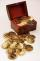Landlord had 49 ducats more than Jurošík. How many ducats Jurošík steal landlord if the Jurošík now 5 ducats more?
• SubtractionTest what do you know about the subtraction of two numbers: a) make a difference if the minuend 4,307,288 and subtrahend is 472008 b) minuend is 4000 more than subtrahend. What's the difference? c) the difference is 38900 less than the minuend. What is th
• Mystery numberA mystery number is between 800 and 920. No digits are the same. The difference of the numbers 5. What is the mystery number?
• Voting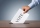400 employees cast their votes in a board member election that has only 2 candidates. 120 people vote for candidate A, while half of the remaining voters abstain. How many votes does candidate B receive?
• DozenWhat is the product of 26 and 5? Write the answer in Arabic numeral. Add up the digits. How many of this is in a dozen? Divide #114 by this
• On the floor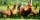You enter the room and there are 2 dogs, 4 horses, 1 giraffe and one duck on the bed, 3 chickens are flying over the chair. How many feet are on the floor?
• Workshop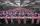In the workshop have to be produced for 5 days 2000 components. How many components must be produced in the workshop every day and how much per hour, if shift is 8 hours.
• Class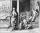When Pythagoras asked how many students attend the school, said: "Half of the students studying mathematics, 1/4 music, seventh silent and there are three girls at school." How many students had Pythagoras at school?
• Street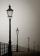Straight streets is long x = 475 meters. At the beginning and at the end of the street are poles. The poles are spaced 25 m. How many poles are on the street?
• Textbooks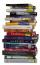After the check of textbooks found that every 10-th textbook should be withdrawn. Together 58 textbooks were withdrawn. How many textbooks were in stock before withdrawn, and how many after withdrawn?
• Unknown number 16My number's tens is three times more then ones My number's ones is twice the number of thousands and my number's hundreds is half the number of of tens. I have two ones. which number am I?
• Students at cinema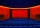All pupils from the school went to the cinema for a film performance. The cinema has 400 seats, 2 rows of 20 seats remain unoccupied. 10 places were occupied by teachers. How many students were there?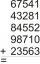Fill letters instead of digits so the indicated sum (equal letters represent equal digits). What number is hidden under the letter J? A A H A H O A H O J -------------------------- 4 3 2 1
• Bus driver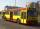The bus driver rides 174 km in the morning, afternoon rides 26 km more. How many kilometers traveled in 5 days if he rides on the same line?
• RemaindersIt is given a set of numbers { 170; 244; 299; 333; 351; 391; 423; 644 }. Divide this numbers by number 66 and determine set of remainders. As result write sum of this remainders.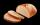They brought in d two kilos and t three kilos of bread to the store. By the end of the day, 14 two-kg bread were sold. How many kilos of bread remained in the store by the next day?
• Brick weightThe brick weighs 2 kg and a half bricks. How much does one brick weigh?
• Alarm clockThe old watchmaker has a unique digital alarm in its collection that rings whenever the sum of digits of the alarm is equal to 21. Find out when the alarm clock will ring. What is their number? List all options . ..
• Dinosaurs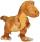More than 30 and less than 60 dinosaurs have met at the pond. A quarter of them bathed and 1/7 saws and the rest gripped. How many were at the pond? How many were there?
• MonkeyThe monkey fell in 40 meters deep well. Every day it climbs 3 meters, at night it dropped back by 2 m. On what day it gets out from the well?

Do you have an interesting mathematical word problem that you can't solve it? Submit a math problem, and we can try to solve it.

We will send a solution to your e-mail address. Solved examples are also published here. Please enter the e-mail correctly and check whether you don't have a full mailbox.

Please do not submit problems from current active competitions such as Mathematical Olympiad, correspondence seminars etc...